# Physics 101 Lecture 21 Waves Physics 101 Lecture

• Slides: 17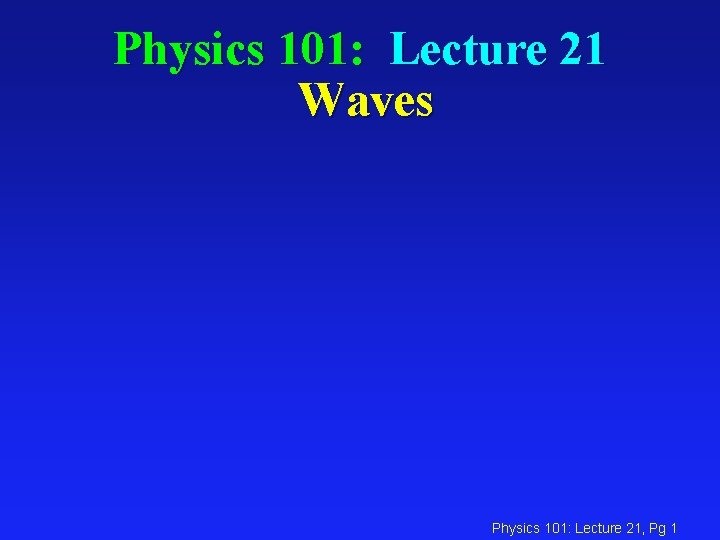Physics 101: Lecture 21 Waves Physics 101: Lecture 21, Pg 1Waves Overview l Types l Speed l Traveling (“harmonic”) l Superposition l Standing Physics 101: Lecture 21, Pg 2 05Types of Waves l Transverse: The medium oscillates perpendicular to the direction the wave is moving. èWater l Longitudinal: The medium oscillates in the same direction as the wave is moving èSound Physics 101: Lecture 21, Pg 3 8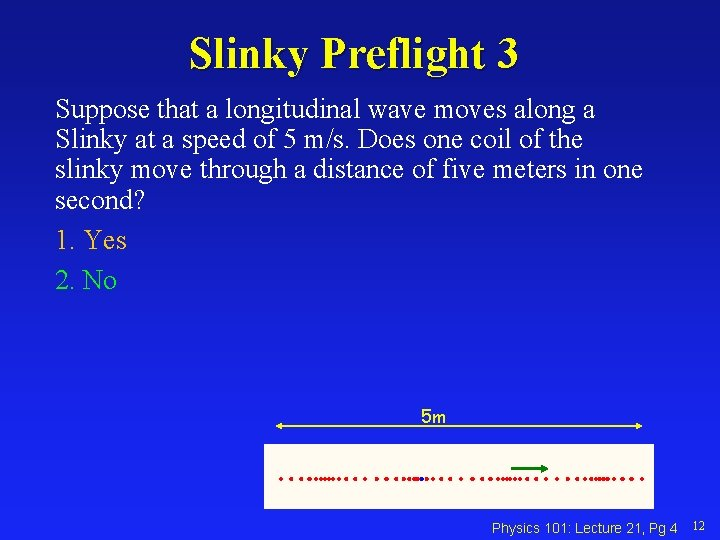Slinky Preflight 3 Suppose that a longitudinal wave moves along a Slinky at a speed of 5 m/s. Does one coil of the slinky move through a distance of five meters in one second? 1. Yes 2. No 5 m Physics 101: Lecture 21, Pg 4 12Velocity of Waves Act A spring and slinky are attached and stretched. Compare the speed of the wave pulse in the slinky with the speed of the wave pulse in the spring. A) vslinky > vspring B) vslinky = vspring C) vslinky < vspring Physics 101: Lecture 21, Pg 5 17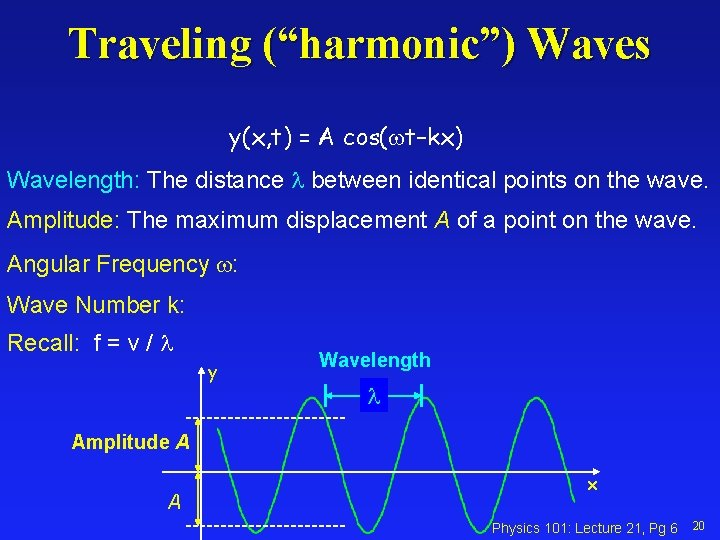Traveling (“harmonic”) Waves y(x, t) = A cos(wt–kx) Wavelength: The distance between identical points on the wave. Amplitude: The maximum displacement A of a point on the wave. Angular Frequency w: Wave Number k: Recall: f = v / y Wavelength Amplitude A A x Physics 101: Lecture 21, Pg 6 20Period and Velocity l l Period: The time T for a point on the wave to undergo one complete oscillation. Speed: The wave moves one wavelength in one period T so its speed is v = / T. f=v/ Physics 101: Lecture 21, Pg 7 22Traveling Waves Exercise y(x, t) = A cos(wt –kx) Label axis and tic marks if the graph shows a snapshot of the wave y(x, t) = 2 cos(4 t – 2 x) at x=0. +2 p/4 -2 p/2 3 p/4 t Physics 101: Lecture 21, Pg 8 25Preflight 1+2 Suppose a traveling wave moves through some medium. If the period of the wave is increased, what happens to the wavelength of the wave assuming the speed of the wave remains the same? 1. The wavelength increases 2. The wavelength remains the same 3. The wavelength decreases Physics 101: Lecture 21, Pg 9 26ACT l The wavelength of microwaves generated by a microwave oven is about 3 cm. At what frequency do these waves cause the water molecules in your burrito to vibrate ? (a) 1 GHz (b) 10 GHz (c) 100 GHz Physics 101: Lecture 21, Pg 10 29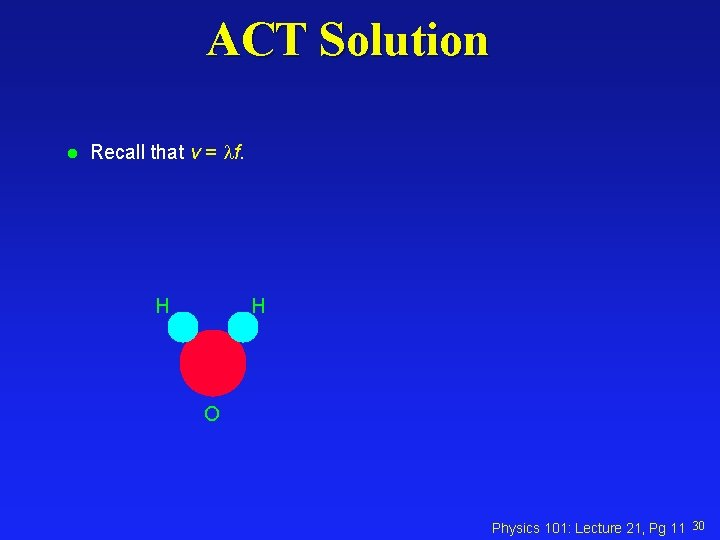ACT Solution l Recall that v = f. H H O Physics 101: Lecture 21, Pg 11 30Visible Absorption coefficient of water as a function of frequency. f = 10 GHz “water window” Physics 101: Lecture 21, Pg 12 31Interference and Superposition l When two waves overlap, the amplitudes add. èConstructive: increases amplitude èDestructive: decreases amplitude Physics 101: Lecture 21, Pg 13 34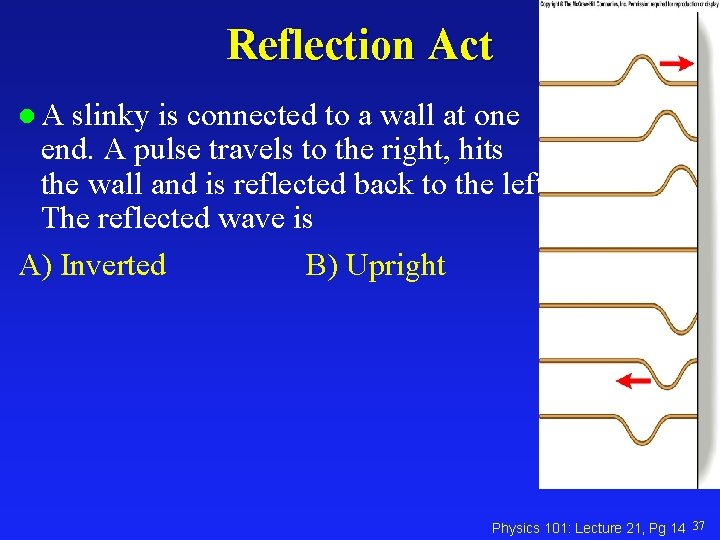Reflection Act l. A slinky is connected to a wall at one end. A pulse travels to the right, hits the wall and is reflected back to the left. The reflected wave is A) Inverted B) Upright Physics 101: Lecture 21, Pg 14 37Standing Waves Fixed Endpoints l Fundamental n=1 (2 nodes) l n = 2 L/n l fn = v/ =n v / (2 L) Physics 101: Lecture 21, Pg 15 44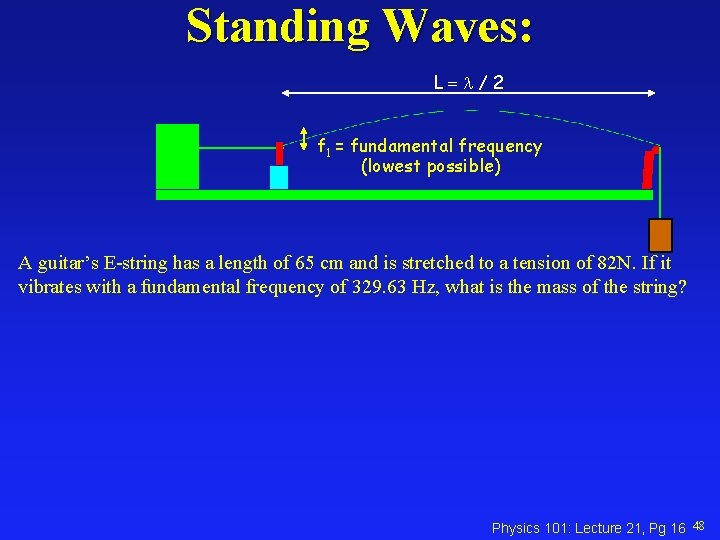Standing Waves: L = / 2 f 1 = fundamental frequency (lowest possible) A guitar’s E-string has a length of 65 cm and is stretched to a tension of 82 N. If it vibrates with a fundamental frequency of 329. 63 Hz, what is the mass of the string? Physics 101: Lecture 21, Pg 16 48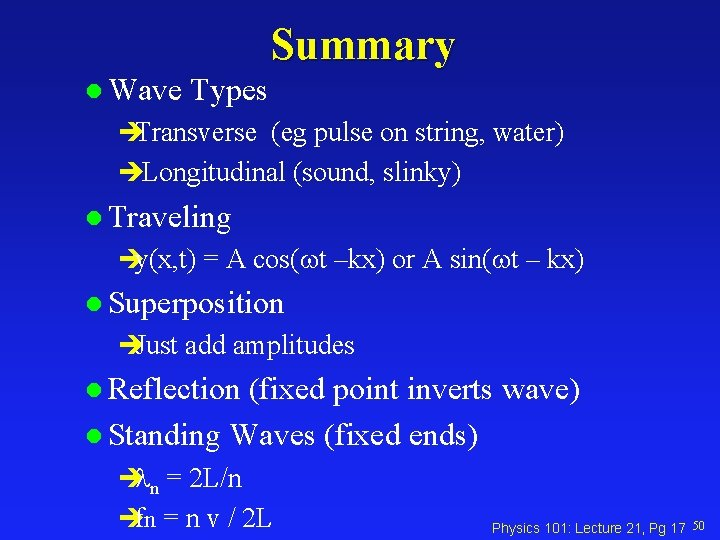Summary l Wave Types èTransverse (eg pulse on string, water) èLongitudinal (sound, slinky) l Traveling èy(x, t) = A cos(wt –kx) or A sin(wt – kx) l Superposition èJust add amplitudes l Reflection (fixed point inverts wave) l Standing Waves (fixed ends) è n = 2 L/n èfn = n v / 2 L Physics 101: Lecture 21, Pg 17 50